Function Repository Resource:

# FunctionDifferentiability

Find the conditions for which a single-variable, real-valued function is differentiable

Contributed by: Wolfram|Alpha Math Team
 ResourceFunction["FunctionDifferentiability"][f,x] returns the conditions for which f is differentiable with respect to x. ResourceFunction["FunctionDifferentiability"][f,{x,n}] returns the conditions for which f is n-times differentiable with respect to x. ResourceFunction["FunctionDifferentiability"][{f,cons},x] returns the conditions for which f constrained to cons is differentiable with respect to x. ResourceFunction["FunctionDifferentiability"][{f,cons},{x,n}] returns the conditions for which f constrained to cons is n-times differentiable with respect to x. ResourceFunction["FunctionDifferentiability"][{{f1,f2,…}},x] returns the conditions for which the functions {f1,f2,…} are all differentiable with respect to x.

## Details

ResourceFunction["FunctionDifferentiability"] has the attribute HoldFirst.
ResourceFunction["FunctionDifferentiability"] takes only real-valued functions.
The constraint cons can be any logical combination of equations or inequalities:
 lhs==rhs equations lhs>rhs,lhs≥rhs,lhs

## Examples

### Basic Examples (3)

Compute the conditions for which a given function is differentiable:

 In:=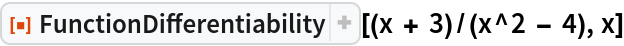Out=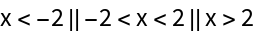Compute the conditions for differentiability of a function subject to a constraint:

 In:=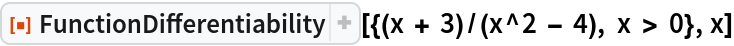Out=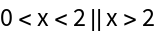A function that is differentiable for all real numbers returns True:

 In:=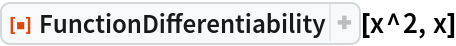Out=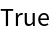The following function is once-differentiable everywhere but not twice-differentiable at x=0:

 In:=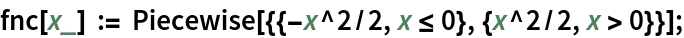In:=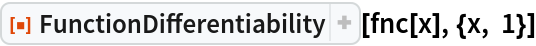Out=In:=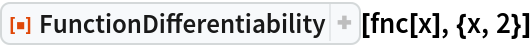Out=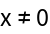### Properties and Relations (2)

FunctionDifferentiability has the attribute HoldFirst, enabling calculations such as the following:

 In:=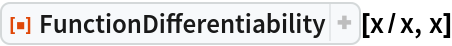Out=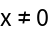For a list of functions, FunctionDifferentiability returns the conditions for which all elements are differentiable:

 In:=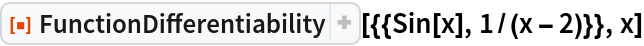Out=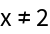### Possible Issues (1)

Since FunctionDifferentiability takes only real-valued functions, it will treat Abs as RealAbs:

 In:=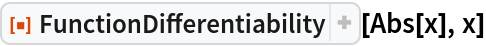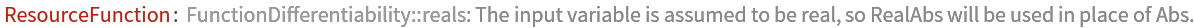Out=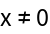## Publisher

Wolfram|Alpha Math Team

## Version History

• 2.0.0 – 23 March 2023
• 1.0.1 – 28 March 2022
• 1.0.0 – 27 July 2021

## Author Notes

To view the full source code for FunctionDifferentiability, evaluate the following:

 In:=